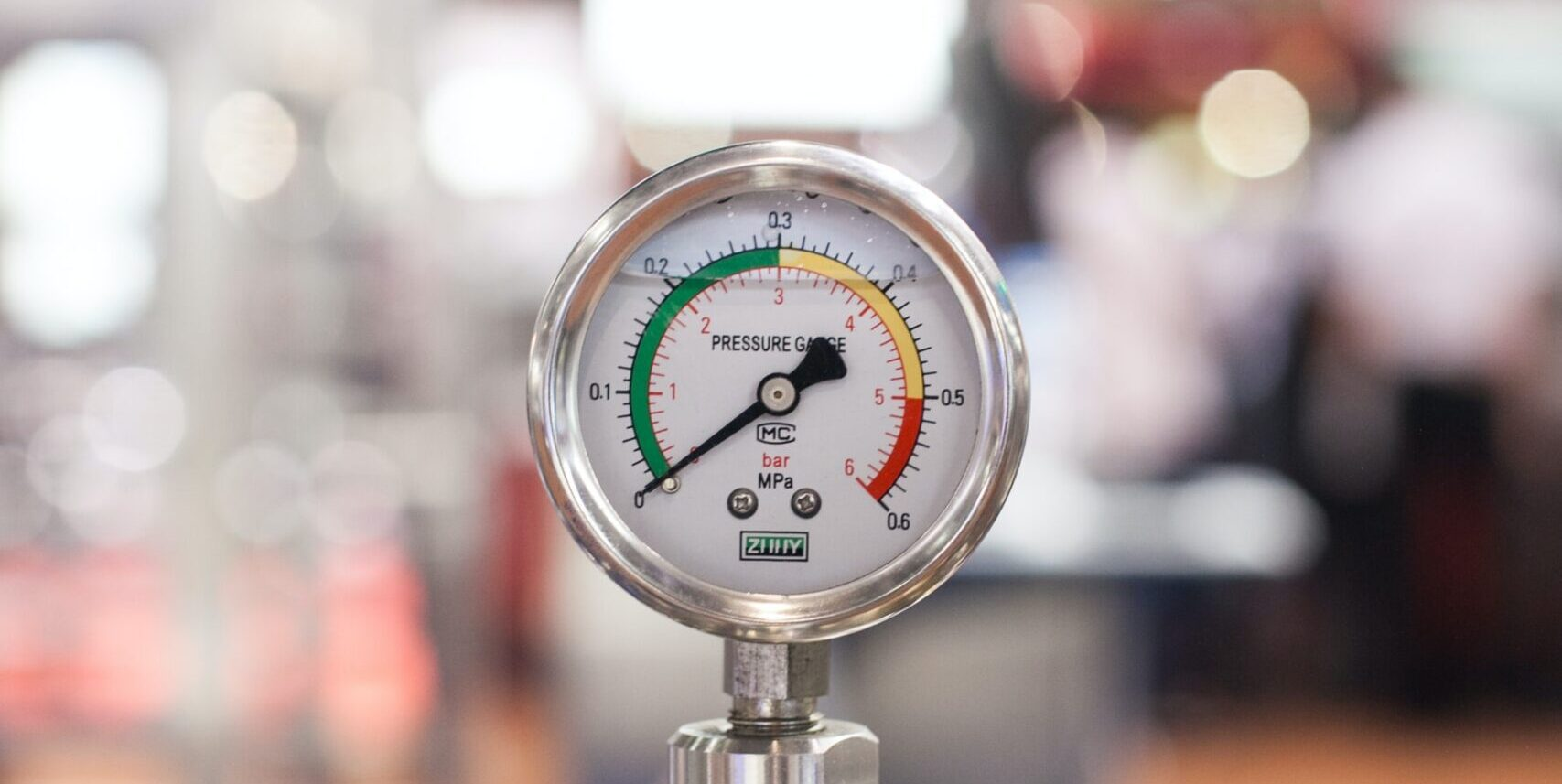# Online Pressure Unit Converter CalculatorPhoto by Crystal Kwok on Unsplash

## What is pressure and how do we measure it?

Pressure is the measure of force or weight over a specified unit of area. Pressure is therefore expressed in units of force or mass (Newtons, kg, ounce etc), divided by units of area (m2, cm2, in2 etc).

Note: force and mass are separate physical parameters, but the relationship between weight and the mass of an object at the surface of the earth is linear, therefore pressure is sometimes defined in terms of mass and not weight.

Use this online pressure unit conversion calculator to easily convert between common units of pressure.

See all of our unit conversion calculators. The area unit conversion tool is based on the pressure unit conversion tables at https://instrumentation.com/.